Example Questions

← Previous 1

Example Question #7 : 45/45/90 Right Isosceles Triangles

An isosceles triangle has a base of 6 and a height of 4. What is the perimeter of the triangle?None of theseExplanation:

An isosceles triangle is basically two right triangles stuck together. The isosceles triangle has a base of 6, which means that from the midpoint of the base to one of the angles, the length is 3. Now, you have a right triangle with a base of 3 and a height of 4. The hypotenuse of this right triangle, which is one of the two congruent sides of the isosceles triangle, is 5 units long (according to the Pythagorean Theorem).

The total perimeter will be the length of the base (6) plus the length of the hypotenuse of each right triangle (5).

5 + 5 + 6 = 16

Example Question #8 : 45/45/90 Right Isosceles Triangles

What is the area of a square that has a diagonal whose endpoints in the coordinate plane are located at (-8, 6) and (2, -4)?

100

100√2

200√2

50

50√2

100

Explanation: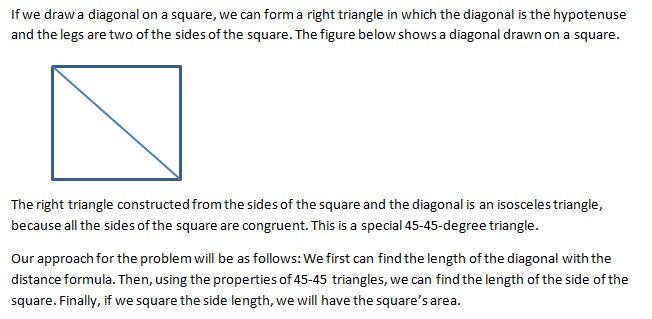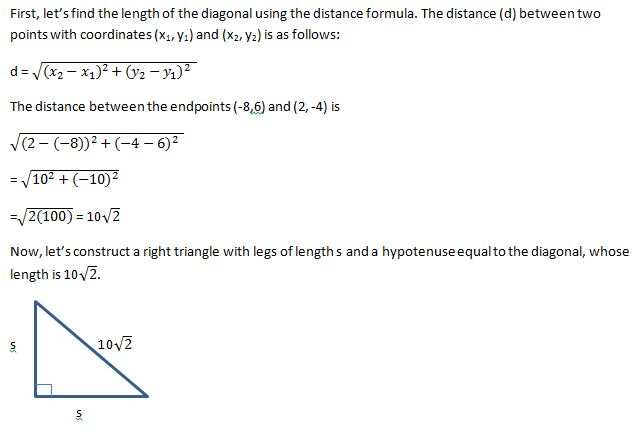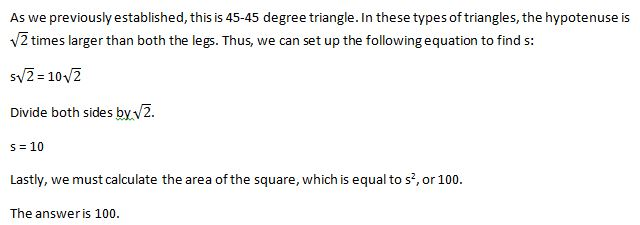Example Question #1 : Isosceles Triangles

The length of the diagonal of a given square is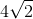. What is the square's area?Explanation:

If we divide the square into two triangles via its diagonal, then we know that the length of the diagonal is equal to the length of the triangles' hypotenuse.

We can use the Pythagorean Theorem to find the length of the two sides of one of our triangles.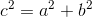Since we're dealing with a square, we know that the two sides of the square (which are the same as the two sides of one of our triangles) will be equal to one another. Therefore, we can say: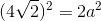Now, solve for the unknown: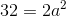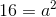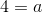This means that the length of the sides of our triangle, as well as the sides of our square, isTo find the area of the square, do the following: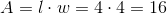.

Example Question #2 : Isosceles Triangles

Two sides of an isosceles triangle are 20 and 30. What is the difference of the largest and the smallest possible perimeters?

0

30

15

10

10

Explanation:

The trick here is that we don't know which is the repeated side. Our possible triangles are therefore 20 + 20 + 30 = 70 or 30 + 30 + 20 = 80.  The difference is therefore 80 – 70 or 10.

Example Question #3 : Isosceles Triangles

A triangle with two equal angles is called a(n) __________.

equilateral triangle

isosceles triangle

disjoint triangle

Pythagoras triangle

right triangle

isosceles triangle

Explanation:

An isoceles triangle is a triangle that has at least two congruent sides (and therefore, at least two congruent angles as well).

Example Question #1 : How To Find An Angle In An Acute / Obtuse Isosceles Triangle

Triangle ABC has angle measures as follows: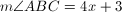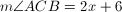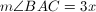What is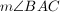?

57

44

90

19

79

57

Explanation:

The sum of the measures of the angles of a triangle is 180.

Thus we set up the equation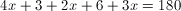After combining like terms and cancelling, we have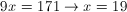Thus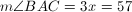Example Question #2 : How To Find An Angle In An Acute / Obtuse Isosceles Triangle

The base angle of an isosceles triangle is five more than twice the vertex angle.  What is the base angle?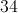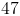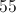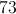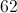Explanation:

Every triangle has 180 degrees.  An isosceles triangle has one vertex angle and two congruent base angles.

Let= the vertex angle and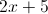= the base angle

So the equation to solve becomes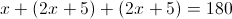Thus the vertex angle is 34 and the base angles are 73.

Example Question #3 : How To Find An Angle In An Acute / Obtuse Isosceles Triangle

The base angle of an isosceles triangle is 15 less than three times the vertex angle.  What is the vertex angle?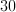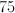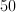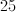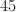Explanation:

Every triangle contains 180 degrees.  An isosceles triangle has one vertex angle and two congruent base angles.

Let= vertex angle and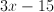= base angle

So the equation to solve becomes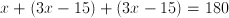.

Example Question #4 : How To Find An Angle In An Acute / Obtuse Isosceles Triangle

The base angle of an isosceles triangle is ten less than twice the vertex angle.  What is the vertex angle?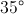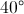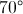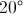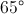Explanation:

Every triangle has 180 degrees.  An isosceles triangle has one vertex angle and two congruent base angles.

Let= vertex angle and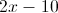= base angle

So the equation to solve becomes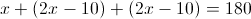So the vertex angle is 40 and the base angles is 70

Example Question #5 : How To Find An Angle In An Acute / Obtuse Isosceles Triangle

The base angle of an isosceles triangle is 10 more than twice the vertex angle.  What is the vertex angle?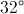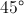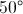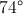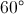Explanation:

Every triangle has 180 degrees.  An isosceles triangle has one vertex angle and two congruent base angles.

Let= the vertex angle and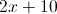= the base angle

So the equation to solve becomes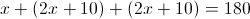The vertex angle is 32 degrees and the base angle is 74 degrees

← Previous 1